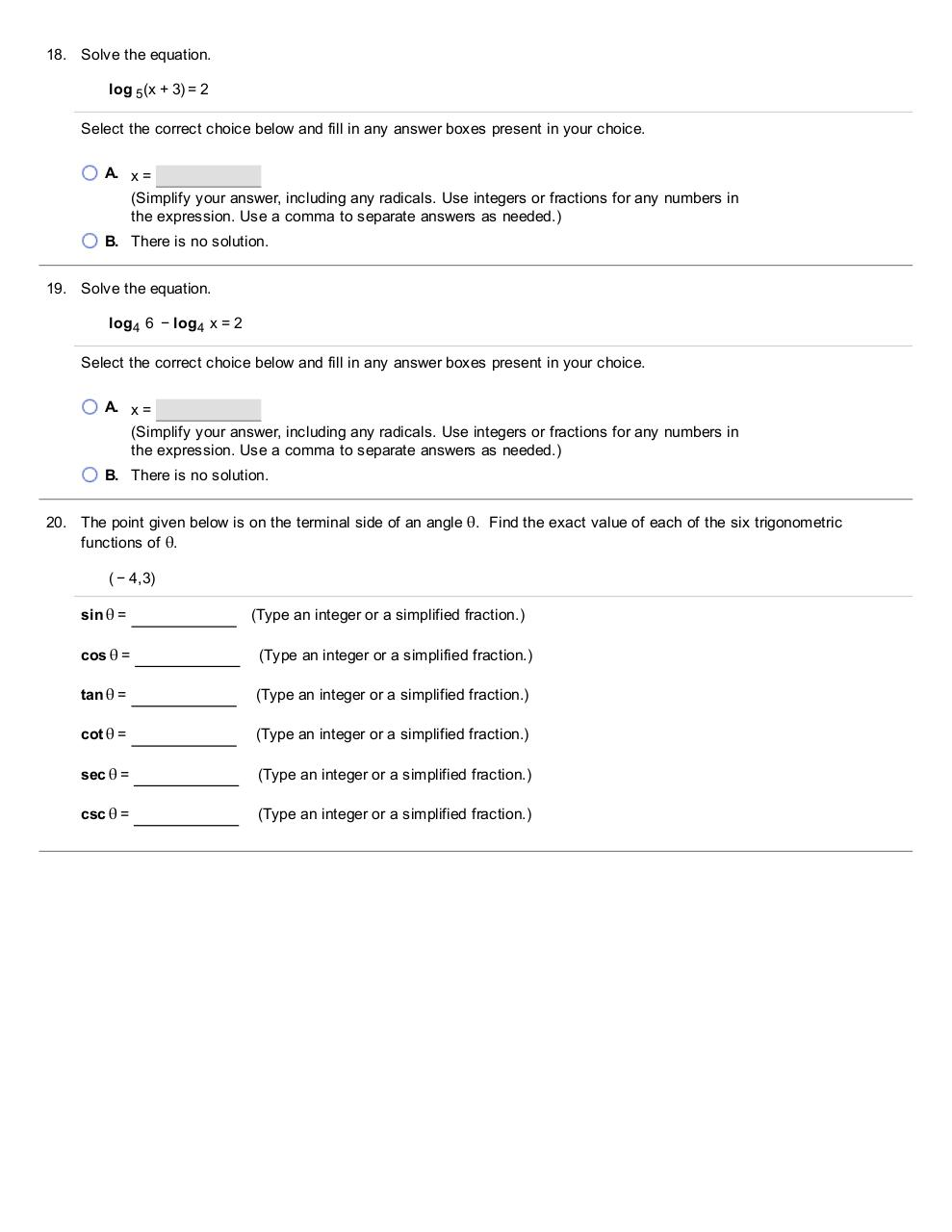# College Level.pdfPage 1 2 3 4 5 6 7 8 9 10

#### Text preview

18. Solve the equation.
log 5 (x + 3) = 2
Select the correct choice below and fill in any answer boxes present in your choice.
A. x =
(Simplify your answer, including any radicals. Use integers or fractions for any numbers in
the expression. Use a comma to separate answers as needed.)
B. There is no solution.
19. Solve the equation.
log4  6  − log4  x = 2
Select the correct choice below and fill in any answer boxes present in your choice.
A. x =
(Simplify your answer, including any radicals. Use integers or fractions for any numbers in
the expression. Use a comma to separate answers as needed.)
B. There is no solution.
20. The point given below is on the terminal side of an angle θ.
Find the exact value of each of the six trigonometric

functions of θ.
( − 4,3)
sin θ =

(Type an integer or a simplified  fraction.)

cos θ =

(Type an integer or a simplified  fraction.)

tan θ =

(Type an integer or a simplified  fraction.)

cot θ =

(Type an integer or a simplified  fraction.)

sec θ =

(Type an integer or a simplified  fraction.)

csc θ =

(Type an integer or a simplified  fraction.)